### Home > CALC > Chapter Ch3 > Lesson 3.1.2 > Problem3-28

3-28.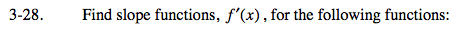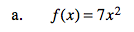Use the Power Rule.If f(x)=axn, then f '(x) = naxn−1

f '(x) = 14x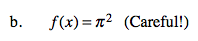Remember that π is just a number, not a variable!

Since π ≈ 3, what is the slope function of y = 32? Use that to find the slope function of f(x) = π2.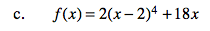f(x) is a horizontal shift of y = 24+18x, whose slope function is easy to find with the Power Rule (see hint in part (a)). As for f(x), THINK! Since slopes will shift with the function... f '(x) will equal a shifted version of y '.

f '(x) = 8(x−2)3+18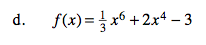See hint in part (a).